## Looking for your content...Type it here

### GATE Previous Years Papers on Instrumentation Engineering

Q. 1 – Q. 20 Carry One Mark Each.

1) Given y = + 2x + 10, the value of is equal to
(A)0
(B) 4
(C) 12
(D)13

2) lim sinx/x
(A) Indeterminate
(B) 0
(C) 1
(D)

3) The power supplied by the dc voltage source in the circuit shown below is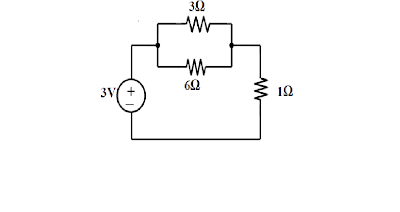(A)0W
(B) 1.0W
(C) 2.5W
(D)3.0W

4) The current I supplied by the dc voltage source in the circuit shown below is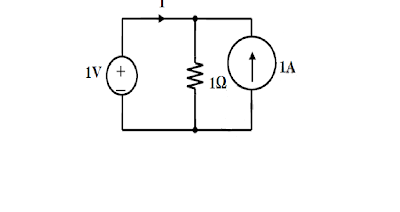(A)0A
(B) 0.5A
(C) 1A
(D)2A

5) For signal conditioning of a piezoelectric type transducer we require
(A) a charge amplifier
(B) a differential amplifier
(C) an instrumentation amplifier
(D) a transconductance amplifier

6) A linear variable differential transformer (LVDT) is
(A) a displacement transducer
(B) an impedance matching transformer
(C) a differential temperature sensor
(D) an auto transformer

7) The temperature being sensed by a negative temperature coefficient (NTC) type thermistor is linearly increasing. Its resistance will
(A) linearly increase with temperature
(B) exponentially increase with temperature
(C) linearly decrease with temperature
(D) exponentially decrease with temperature

8) For a single stage BJT common base amplifier
(A) current gain as well as voltage gain can be greater than unity
(B) current gain can be greater than unity but voltage gain is always less than unity
(C) voltage gain can be greater than unity but current gain is always less than unity
(D) current gain as well as voltage gain is always less than unity

9) In the circuit shown below, the ideality factor of the diode is unity and the voltage drop across it is 0.7V. The dynamic resistance of the diode at room temperature is approximately(A)15 Ω
(B) 25 Ω
(C) 50 Ω
(D)700 Ω

10) An ideal op-amp has the characteristics of an ideal
(A) voltage controlled voltage source
(B) voltage controlled current source
(C) current controlled voltage source
(D) current controlled current source

11) The inverters in the ring oscillator circuit shown below are identical. If the output waveform has a frequency of 10 MHz, the propagation delay of each inverter is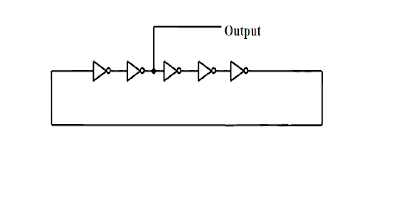(A) 5 ns
(B) 10 ns
(C) 20 ns
(D) 50 ns

12) A 2K×8 bit RAM is interfaced to an 8-bit microprocessor. If the address of the first memory location in the RAM is 0800H, the address of the last memory location will be
(A)1000H
(B) 0FFFH
(C) 4800H
(D)47FFH

13) The fundamental period of the discrete-time signal is

14) Which one of the following discrete-time systems is time invariant?

15) If a current of A is passed through a true RMS ammeter, the meter reading will be
(A)6A
(B) A
(C) 12A

16) If the ac bridge circuit shown below is balanced, the element Z can be a(A)Pure capacitor
(B) Pure inductor
(C) RL series combination
(D)RL parallel combination

17) The Bode asymptotic plot of a transfer function is given below. In the frequency range shown, the transfer function has(A) 3 poles and 1 zero
(B) 1 pole and 2 zeroes
(C) 2 poles and 1 zero
(D) 2 poles and 2 zeroes

18) For radioisotope imaging, an Anger camera is fitted with a parallel hole collimator. If the thickness of the collimator is increased, the camera
(A) resolution and sensitivity will increase
(B) resolution and sensitivity will decrease
(C) resolution will increase and sensitivity will decrease
(D) resolution will decrease and sensitivity will increase

19) In the standard 12lead ECG recording system, the minimum number of electrodes required to be attached to a human subject for recording any one of the unipolar chest lead signals is
(A)1
(B) 2
(C) 4
(D)5

20) A laser light with a wave length of 633nm is passed through 1cm length of tissue and 2cm length of glass. The refractive indices of tissue and glass are 1.33 and 1.5 respectively. The velocities of laser light in the tissue and in the glass are in the ratio of
(A) 1.33 : 0.75
(B) 1.33 : 3.0
(C) 1.33 : 1.5
(D) 1.5 : 1.33

Q. No. 21 to 75 Carry Two Marks Each

21) The expression for is equal to

22) Consider the differential equation . Which one of the following can be a particular solution of this differential equation?

23) Consider the function . The maximum value of y obtained when x varies over the interval 2 to 5 is
(A)1
(B) 3
(C) 4
(D)9

24) It is known that two roots of the nonlinear equation 6 are 1 and 3.The third root will be
(A) j
(B) – j
(C) 2
(D)4

25) Consider a Gaussian distributed random variable with zero mean and standard deviation . The value of its cumulative distribution function at the origin will be
(A)0
(B) 0.5
(C) 1
(D)10

26) A random variable is uniformly distributed over the interval 2 to 10. Its variance will be
(A)
(B) 6
(C)
(D)36

27) The Fourier transform of , where u(t) is the unit step function,
(A)Exists for any real value of a
(B)Does not exist for any real value of a
(C) Exists if the real value of a is strictly negative
(D)Exists if the real value of a is strictly positive

28) In the circuit shown below the maximum power that can be transferred to the load is
i(t) = 10 sin (1000t)A(A)250W
(B) 500W
(C) 1000W
(D)2000W

29) A complete variable Z = x + j0.1 has its real part x varying in the range ∞ to ∞. Which one of the following is the locus (shown in thick lines) of 1/Z in the complex plane?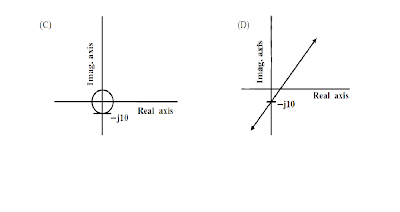30) For the circuit shown below the input resistance is(A) 3Ω
(B) 2Ω
(C) 3Ω
(D)13Ω

31) In the circuit shown below the average power consumed by the 1Ω resistor is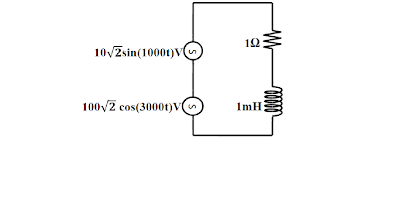(A)50W
(B) 1050W
(C) 5000W
(D)10100W

32) Which one of the following equations is valid for the circuit shown below?33) For the circuit shown below the steady-state current I is
v(t) = 5cos(1000t)V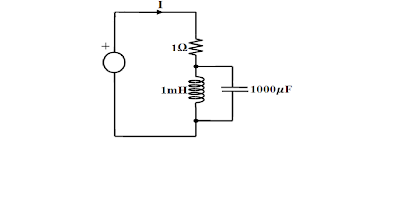(A)0A
(B) 5 cos(1000t)A
(C) A
(D)5 A

34) For the circuit shown below the voltage across the capacitor is(A) (10 + j0)V
(B) (100 + j0)V
(C) (0 + j100)V
(D) (0 j100)V

35) The speed of a gear having 60 teeth is measured using a proximity sensor. The output of the proximity sensor is fed to a counter with a gating time of 1s. The counter indicates a value of 120. The speed at which the gear is rotating is
(A) 60 rpm
(B) 120 rpm
(C) 600 rpm
(D) 1200 rpm

36) A piezoelectric type accelerometer has a sensitivity of 100mV/g. The transducer is subjected to a constant acceleration of 5g. The steady state output of the transducer will be
(A)0V
(B) 100mV
(C) 0.5V
(D)5V

37) A pair of identical thermocouples is employed for measuring the temperature of a specimen as shown below. The characteristic of the thermocouples is tabulated below. The reference junction is at 2°C. The meter reads 48V . The correct temperature of the specimen isTemperature() 0 10 20 30 40 50 60 70 80 90 Output() 35 45 55 65 75 85 95 105 115 125
(A)13
(B) 46
(C) 48
(D)50

38) A strain gauge has a nominal resistance of 600Ω and a gauge factor of 2.5. The strain gauge is connected in a dc bridge with three other resistances of 600Ω each. The bridge is excited by a 4V battery. If the strain gauge is subjected to a strain of 100 m/m, the magnitude of the bridge output will be

(A)0V
(B) 250μV
(C) 500μV
(D)750μV

39) The torque in a rotating shaft is measured using strain gauges. The strain gauges must be positioned on the shaft such that the axes of the strain gauges are at

(A) with respect to the axis of the shaft
(B) 3with respect to the axis of the shaft
(C) 4 with respect to the axis of the shaft
(D) 9 with respect to the axis of the shaft

40) To reduce the effect of fringing in a capacitive type transducer

(A) the transducer is shielded and the shield is kept at ground potential
(B) a guard ring is provided and it is kept at ground potential
(C) the transducer is shielded and the shield is kept at the same potential as the moving plate
(D) a guard ring is provided and it is kept at the same potential as the moving plate

41) A differential amplifier shown below has a differential mode gain of 100 and a CMRR of 40dB. If(A)10V
(B) 10.5V
(C) 11V
(D)15V

42) The op-amp circuit shown below is that of a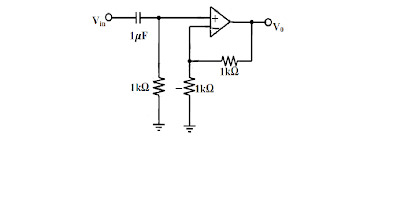(A) low-pass filter with a maximum gain of 1
(B) low-pass filter with a maximum, gain of 2
(C) high-pass filter with a maximum gain of 1
(D) high-pass filter with a maximum gain of 2

43) In the op-amp circuit shown below the input voltage vin is gradually increased from 10V to +10V. Assuming that the output voltage vout saturates at 10V and +10V,vout will change from(A) 10V to +10V when vin
(B) 10V to +10V when v 1V
in
(C) +10V to 10V when v 1V
in
(D)+10V to 10V when v 1V
in 1V

44) For the op-amp circuit shown below v 1V 0 is approximately equal to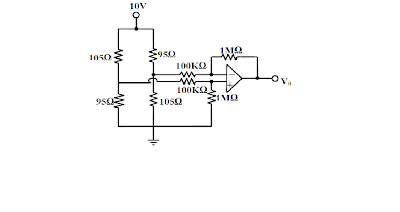(A) 10V
(B) 5V
(C) +5v
(D)+10V

45) In the amplifier circuit shown below, assume VBE = 0.7V and the β of the transistor and the values of C1 and C2 are extremely high. If the amplifier is designed such that at the quiescent point its VCE = where VCC is the power supply voltage, its small signal voltage gain will be(A) 3.75
(B) 4.5
(C) 9
(D)19

46) The result of (45)10 – (45)16 expressed in 6bit 2’s complement representation is

(A)011000
(B) 100111
(C) 101000
(D)101001

47) The output F of the multiplexer circuit shown below expressed in terms of the inputs P, Q and R is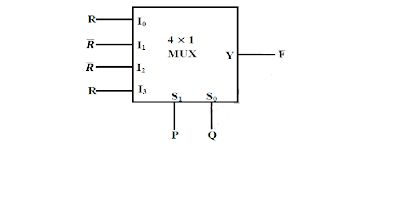(A)F = PQR
(B) F = PQ+QR+RP
(C) F = (P Q)R
(D)F = (PQ)

48) A part of a program written for an 8085 microprocessor is shown below. When the program execution reaches LOOP2, the value of register C will be
SUB A
MOV C, A
LOOP 1: INR A
DAA
JC LOOP2
INR C
JNC LOOP1
LOOP2: NOP

(A)63H
(B) 64H
(C) 99H
(D)100H

49) 49. The minimum sum of products form of the Boolean expression
Y= +P +P S +P R S +P + is
(A)Y = P
(B)Y = P
(C) Y = P
(D)Y = +PR

50) An X-ray tube is operated at 80kV anode voltage. In order to filter the low intensity X-rays, a 2.5mm thick aluminum filter is used. It is given that at 80 kV anode voltage, the mass attenuation coefficients and densities of aluminum are 0.02 and 2699 Kg respectively and for copper these are 0.075 and 8960 Kg respectively. If a copper filter is to replace the aluminum filter with the same filtering effect, the thickness of the copper filter should b

(A)0.2mm
(B) 0.66mm
(C) 1.5mm
(D)5mm

51) A 5 MHz acoustic pulse travels from a transducer through a 2cm thick fat tissue before it encounters an interface with a liver tissue at normal incidence. The amplitude attenuation factors of fat and liver are 0.075Np/MHz and 0.1Np/MHz respectively. The amplitude reflectivity coefficient of fat-liver interface is 0.1. Taking both attenuation and reflection losses into account, the amplitude loss (in dB) of echo pulse when it returns to the transducers is

(A) 0.74
(B) 2.6
(C) 6
(D) 33

52) Consider a discrete-time LTI system with input x[n] = [n][n-1] and impulse response h[n] =[n][n1]. The output of the system will be given by
(A)
(B)
(C)
(D)

53) Consider a discrete-time system for which the input x[n] and the output y[n] are related as .If y[n] = 0 for n < fc =" 2MHz"> 2
(B) <>

58) The step response of a linear time invariant system is , where u(t) is the unit step function. If the output of the system corresponding to an impulse input (t) is h(t), then h(t) is
(A)
(B)
(C)
(D)

59) A 2A full-scale PMMC type dc ammeter has a voltage drop of 100 mV at 2A. The meter can be converted into a 10A full-scale dc ammeter by connecting a

(A) 12.5 mΩ resistor in parallel with the meter
(B) 12.5 mΩ resistor in series with the meter
(C) 50.0 mΩ resistor in parallel with the meter
(D) 50.0 mΩ resistor in series with the meter

60) A 3½ digit, 200 mV full scale DVM has an accuracy specification of 0.5% of reading plus 5 counts. When the meter reads 100 mV, the voltage being measured is

(A)Any value between 99.5 mV and 100.5 mV
(B)Any value between 99.0 mV and 101.0 mV
(C) Exactly 99.5 mV
(D)Exactly 100 mV

61) A 230 V, 5A, 50 Hz single phase house service energy meter has a meter constant of 360rev/kWhr. The meter takes 50s for making 51 revolutions of the disc when connected to a 10 kW, unity power factor load. The error in the reading of the meter is
(A)0%
(B) +0.5%
(C) 2.0%
(D)+2.0%

62) The op-amp based circuit of a half wave rectifier electronic voltmeter shown below uses a PMMC ammeter with a full scale deflection (FSD) current of 1 mA and a coil resistance of 1kΩ . The value of R that gives FSD for a sinusoidal input voltage of 100 mV (RMS) is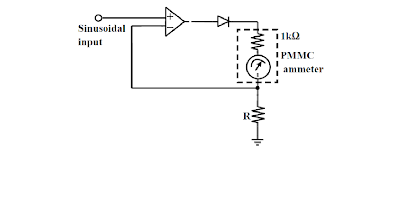(A)45Ω
(B) 67.5 Ω
(C) 100Ω
(D)144.4 Ω

63) The x and y sensitivities of an analog oscilloscope are set as 2 ms/cm and 1V/cm respectively. The trigger is set at 0V with negative slope. An input of 2cos (100t + )V is fed to the y input of the oscilloscope. The wave form seen on the oscilloscope will be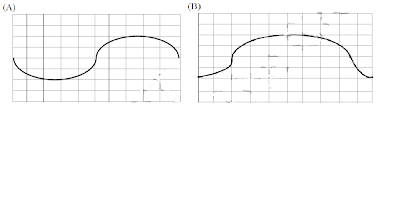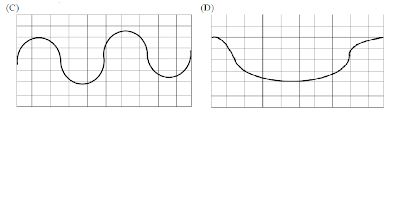64) The open loop transfer function of a unity feedback system is G(s) = . The root locus plot of the system has

(A) two breakaway points located at and
(B) one breakaway point located at
(C) one breakaway point located at
(D)one breakaway point located at

65) If a first order system and its time response to a unit step input are as shown below, the gain K is(A) 0.25
(B) 0.8
(C) 1
(D)4

66) The state space representation of a system is given by
The transfer function of the system will be
(A)
(B)
(C)
(D)

67) A closed loop control system is shown below. The range of the controller gain which will make the real parts of all the closed loop poles more negative than 1 is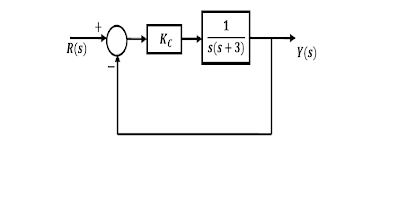(A)
(B) > 0
(C) > 2
(D) < href="http://1.bp.blogspot.com/-fcYYlGjz5pA/TYieX9PziMI/AAAAAAAAAek/AwIR0_tPIIQ/s1600/Untitled67.png">(A)
(B)
(C)
(D)

69) A tissue with a refractive index 1.33 is introduced in one of the light paths of a Michelson interferometer operating with a monochromatic coherent light source of wavelength 589nm. After the introduction of a tissue sample of thickness t, the fringe pattern is observed to shift by 50 fringes. If the thickness is 2t , the fringe pattern will shift by

(A) 25 fringes
(B) 50 fringes
(C) 100 fringes
(D) 200 fringes

70) In the process of non-destructive testing of a 10cm diameter cylinder, a cross-sectional (transaxial) image of the cylinder is reconstructed with the help of parallel beam computer tomography technique. To realize a spatial resolution of 1mm in the image, the minimum number of ray samples in each projection set and the minimum number of projection sets required are

(A) 200 and 315 respectively
(B) 100 and 315 respectively
(C) 200 and 629 respectively
(D) 100 and 629 respectively

Common Data Questions

Common Data Questions 71, 72 & 73:

A data acquisition system (DAS) shown below employs a successive approximation type 12-bit ADC having a conversion time of 5 μs.71) The quantization error of the ADC is
(A)0%
(B) 0.012%
(C) 0.024%
(D) 0.048%

72) The system is used as a single channel DAS with channel 1 selected as input to the ADC which is set in the continuous conversion mode. For avoiding aliasing error, the cutoff frequency of the filter in channel 1 should be

(A) 100kHz
(B) 100kHz
(C) 100kHz < style="font-weight: bold;">Common Data Questions:

74 & 75 Laser light is generated by energizing helium-neon gas in a chamber. The ground and metastable states of helium are 0 eV and 20.61 eV respectively. The ground, higher and metastable energies of neon are 0 eV, 18.70 eV and 20.66 eV respectively. The values of speed of light, Planck constant and charge of electron are 3 m/ s, 6.625 Js and 1.6 respectively

74) In this process, helium molecules

(A) play no role
(B) produce laser light
(C) give energy to neon molecules
(D) absorb energy from neon molecules

75) Wavelength of laser light generated in this process is

(A) 61.6nm
(B) 66.4nm
(C) 633.8nm
(D) 650.3nm

Q.76 to Q.85 Carry Two Marks Each Statement for Linked Answer Questions: 76 & 77

In the Wheatstone bridge shown below the galvanometer G has a current sensitivity of 1μA /mm, a resistance of 2.5kΩ and a scale resolution of 1 mm. Let R be the minimum increase in R from its nominal value of 2kΩ that can be detected by this bridge.76) When R is 2kΩ + R, is

(A)6 V
(B) 6.0024 V
(C) 6.0038V
(D) 6.005 V

77) The value of R is approximately

(A)2.8 Ω
(B) 3.4 Ω
(C) 5.2 Ω
(D)12 Ω

In the circuit shown below the steady-state is reached with the switch K open. Subsequently the switch is closed at time t = 0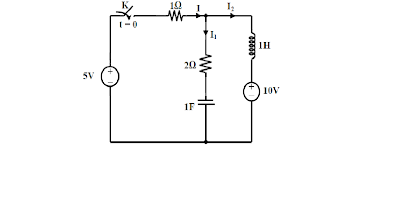78) At time t = , current I is
(A)
(B) 0A
(C)
(D) ∞ A

79) At time t = , is

(A) 5A / s
(B)
(C) 0A / s
(D) 5A / s

Consider the counter circuit shown below.80) In the above figure, Y can be expressed as

81) The above circuit is a

(A)Mod – 8 Counter
(B) Mod – 9 Counter
(C) Mod – 10 Counter
(D)Mod – 11 Counter

Consider a unity feedback system with open loop transfer function

82) The phase crossover frequency of the system in radians per second is
(A) 0.125
(B) 0.25
(C) 0.5
(D) 1

83. The gain margin of the system is
(A) 0.125
(B) 0.25
(C) 0.5
(D) 1

A unity feedback system has open loop transfer function . The time at which the response to a unit step input reaches its peak is seconds.

84) The damping coefficient for the closed loop system is
(A) 0.4
(B) 0.6
(C) 0.8
(D) 1

85) The value of p is
(A) 6
(B) 12
(C) 14
(D) 16